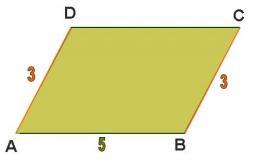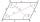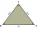# Parallelogram

Calculate the missing side of a parallelogram, if you know the perimeter o and one side:

a) o = 7.2 cm; b = 1.8 cm
b) o = 5.4 cm; a = 1.9 cm

Correct result:

a =  1.8 cm
b =  0.9 cm

#### Solution:We would be pleased if you find an error in the word problem, spelling mistakes, or inaccuracies and send it to us. Thank you!#### You need to know the following knowledge to solve this word math problem:

We encourage you to watch this tutorial video on this math problem:

## Next similar math problems:

• IronIron ore contains 57% iron. How much ore is needed to produce 20 tons of iron?
• CircleWhat is the radius of the circle whose perimeter is 6 cm?
• HotelThe hotel has a p floors each floor has i rooms from which the third are single and the others are double. Represents the number of beds in hotel.
• FlowerbedIn the park there is a large circular flowerbed with a diameter of 12 m. Jakub circulated him ten times and the smaller Vojtoseven times. How many meters each went by and how many meters did Jakub run more than Vojta?
• Simple equationsSolve system of equations: 5x+3y=5 5x+7y=25
• GivenGiven 2x =0.125 find the value of x
• Clock handsThe second hand has a length of 1.5 cm. How long does the endpoint of this hand travel in one day?
• Circle - simpleThe circumference of a circle is 198 mm. How long in mm is its diameter?
• ParallelogramIn the parallelogram we know one internal angle 67°33`. Calculate the other internal angles.
• Perimeter to areaCalculate the area of a circle with the perimeter 15 meters.
• Simplify 2Simplify expression: 5ab-7+3ba-9
• Find theFind the radius of the circular base of the vessel, whose perimeter is 2.51 m. Write the result to one decimal place in meters. Via the π sign - Ludolph's number
• Arm-legCalculate the length of the base of an isosceles triangle with a circumference 224 cm if the arm length is 68 cm.
• Sides of triangleTriangle circumference with two identical sides is 117cm. The third side measures 44cm. How many cms do you measure one of the same sides?
• Circle r,DCalculate the diameter and radius of the circle if it has length 26.59 cm.
• Four circles1) Calculate the circle radius if its area is 400 cm square 2) Calculate the radius of the circle whose circumference is 400 cm. 3) Calculate circle circumference if its area is 400 cm square 4) Calculate the circle's area if perimeter 400 cm.
• TrianglesEquilateral triangle with side 40 cm has the same perimeter as an isosceles triangle with arm of 45 cm. Calculate the base x of an isosceles triangle.